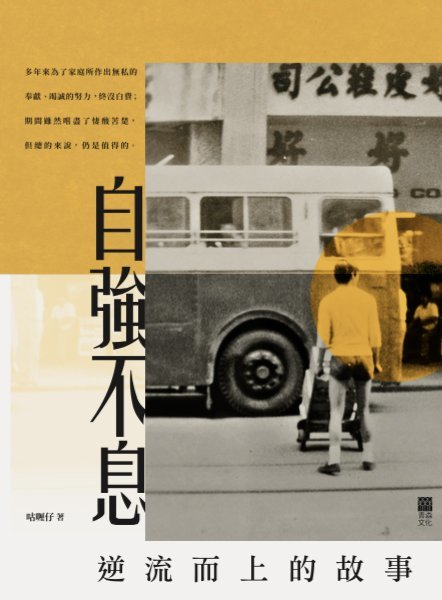# 自強不息——逆流而上的故事

• 紙書

### 咕喱仔

• 目錄
• （一）奮鬥人生由此起
• （二）新嚟必成新豬肉
• （三）聲色犬馬情深厚
• （四）咕喱生涯何其苦
• （五）加班送貨得人驚
• （六）神級車禍天庇佑
• （七）半條人命命坎坷
• （八）人民內部真矛盾
• （九）考牌難過考狀元
• （十）散倉特警冇乜景
• （十一）柴娃娃公司旅行
• （十二）梳打埠攪笑紀行
• （十三）調職超市開荒牛
• （十四）嚴父愚子喜或悲
• （十五）瘦譚無奈也移民
• （十六）石梨家訪敢死隊
• （十七）黐黐呆呆坐一枱
• （十八）另尋工作為讀書
• （十九）清貧窮苦原是罪
• （二十）奮力衝出起跑線
• （二十一）榮任正牌大嘥鬼
• （二十二）匪夷所思的家庭
• （二十三）情竇初開小姑娘
• （二十四）情同手足話當年
• （二十五）自我放逐看世情
• （二十六）重整旗鼓換新顏
• （二十七）變幻從來是永恆
• （二十八）狗馬的傳奇人生
• （二十九）數年人事盡變遷
• （三十）真心至誠的道別
• （三十一）夜校生活的哀歌
• （三十二）念念不忘亦要忘
• （三十三）山雨欲來風滿樓
• （三十四）清貧窮苦仍是罪
• （三十五）學習等待與忍耐
• （三十七）瞬間搶佔橋頭堡
• （三十八）盡全力鞏固成果
• （三十九）永遠永遠懷念你
• （四十）另類管理方程式
• （四十一）白米發霉都照擸
• （四十二）天下無不散筵席
• （四十三）各有各的價值觀
• （四十四）新的跑道新希望
• （四十五）天下烏鴉一樣黑
• （四十六）一盤散沙愛爭鬥
• （四十七）雖曾苦盼輕放手
• （四十八）芸芸眾生鬥莫停
• （四十九）出爾反爾難立信
• （五十）破格調職文憑組
• （五十一）據理力爭不退讓
• （五十二）臨時考古從業員
• （五十三）培訓部首台電腦
• （五十四）榮升摩登有巢士
• （五十五）八年打拼終圓夢
• （五十六）繼續進修莫停息
• （五十七）新聘南洋大學生
• （五十八）不可理喻的族群
• （五十九）祈創幸福小天地
• （六十）無辜誤墮眼鏡黨
• （六十一）黃粱夢醒緣已盡
• （六十二）紙紮下巴口輕輕
• （六十三）一貧如洗的歲月
• （六十四）覆水難收情難再
• （六十五）世上自有留人地
• （六十六）處處無家處處家
• （六十七）萍水相逢情非淺
• （六十八）神奇女俠挽狂瀾
• （六十九）離合聚散常變遷
• （七十）一人一屋一個家
• （七十一）死神再次開小差
• （七十二）賣屋買屋再搬屋
• （七十三）如願以償樂轉崗
• （七十四）當時得令白草油
• （七十五）投機炒賣樂趣多
• （七十六）施展奇謀奪佳績
• （七十七）人生何處不相逢
• （七十八）愧無面目再相見
• （七十九）再接再厲取文憑
• （八十）學成歸來鬼見愁
• （八十一）心猿意馬芳心亂
• （八十二）野草嬌花諧鸞鳳
• （八十三）家族體面千斤重
• （八十四）羽翼既成展翅飛
• （八十五）有自唔在攞苦辛
• （八十六）海上驚魂暴風狂
• （八十七）高手過招風雲變
• （八十八）惺惺相惜在微時
• （八十九）義助下屬展新篇
• （九十）絕世神功A字膊
• （九十一）養子方知父母恩
• （九十二）慈母愚子好夥伴
• （九十三）金融風暴損失重
• （九十四）火紅年代狂眾生
• （九十五）發配邊疆再轉型
• （九十六）深耕細作謀改善
• （九十七）全面融入拒違規
• （九十八）摩登陸榮廷睇相
• （九十九）提升形象取認證
• （一零零）退休制度胡亂改
• （一零一）常理化建議奪奬
• （一零二）科網股爆破慘案
• （一零三）接掌帥印任翱翔
• （一零四）學歷銜頭不值錢
• （一零五）嚴正使用酌情權
• （一零六）為人父母你我他
• （一零七）路見不平必相助
• （一零八）現身說法談管理
• （一零九）大限臨頭各顧各
• （一一零）經濟動物嬌滴滴
• （一一一）人在胡鬧天在看
• （一一二）勾心鬥角誰之過
• （一一三）小孩成長靠父母
• （一一四）一入侯門深似海
• （一一五）男兒有淚不輕彈
• （一一六）本想忘懷又記起
• （一一七）領導無方眾別離
• （一一八）奇蹟雖現亦難留
• （一一九）成就指標重財富
• （一二零）沉溺炒賣已瘋狂
• （一二一）已盡全力誰珍惜
• （一二二）迫虎跳牆莫奈何
• （一二三）衷誠合作無忌諱
• （一二四）金融海嘯愁煞人
• （一二五）升中派位費苦心
• （一二六）敢於堅持償所願
• （一二七）既不成功欲成仁
• （一二八）逃責托孤仲夏夜
• （一二九）狠心決絕胡不歸
• （一三零）任意妄為誰受苦
• （一三一）擦乾淚水再上路
• （一三二）二次傷害情何堪
• （一三三）亡妻設靈百感生
• （一三四）緣分雖斷情難斷
• （一三五）重拾勇氣盡承擔
• （一三六）稱職父親不易為
• （一三七）重返家園情不再
• （一三八）敞開心窗盡釋懷
• （一三九）憑歌寄意訴衷情
• （一四零）新的生活新的夢
• （一四一）物競天擇適者存
• （一四二）家家有本難唸經
• （一四三）望穿秋水終退休
• （一四四）王子公主皆生病
• （一四五）生活壓力何其多
• （一四六）生不如死論隱青
• （一四七）逃出凡籠奮力飛
• （一四八）義助煎藥福與禍
• （一四九）為誰辛苦為誰忙
• （一五零）誓取金榜提名時
• （一五一）父子同心能斷金
• （一五二）成績尚可選科忙
• （一五三）亡妻夙願終能償
• （一五四）人生成長難駕馭
• （一五五）世代撕裂誰主導
• （一五六）皇天不負有心人
• （一五七）桃花依舊笑春風
• （一五八）迷途知返誠可貴
• （一五九）廣島四天全包團
• （一六零）一個甲子的反思